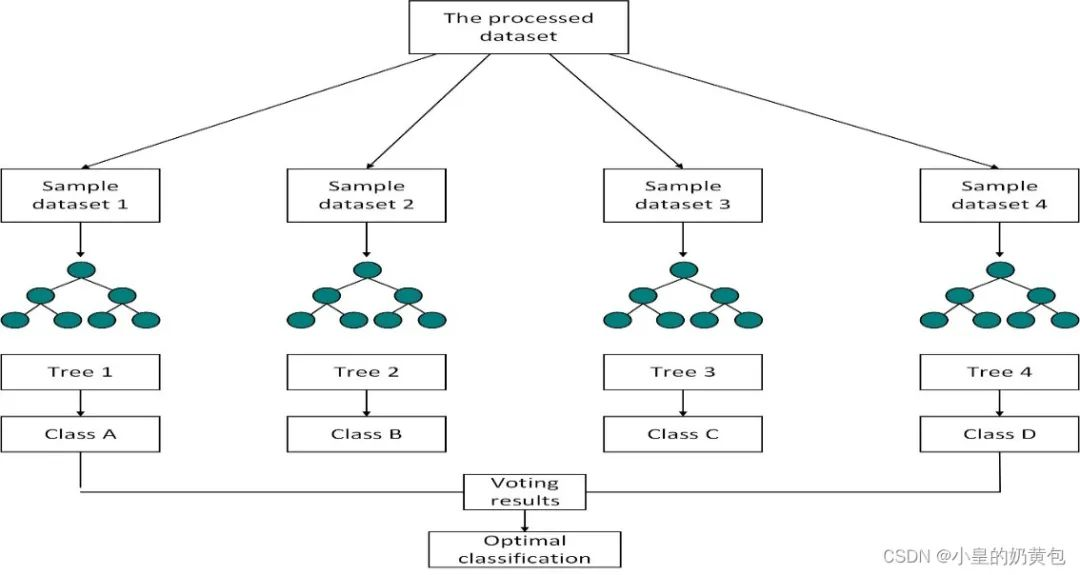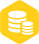# 基于Python实现随机森林算法

### 描述

0 绪论

Python编译器：Pycharm社区版或个人版等Figure 1  随机森林分类流程图

3.1 数据加载

```import pandas as pd
# 加载数据

3.2 创建随机森林分类器

```from sklearn.ensemble import RandomForestClassifier
# 创建随机森林分类器
clf = RandomForestClassifier(n_estimators=100)```

3.3 创建ShuffleSplit对象，用于执行自动洗牌

```from sklearn.model_selection import ShuffleSplit
# 创建ShuffleSplit对象，用于执行自动洗牌
ss = ShuffleSplit(n_splits=1, train_size=0.7, test_size=0.3, random_state=0)```

3.4 循环遍历每个拆分，并使用随机森林分类器对每个拆分进行训练和评估

```from sklearn.metrics import confusion_matrix, classification_report, accuracy_score
# 循环遍历每个拆分，并使用随机森林分类器对每个拆分进行训练和评估
for train_index, test_index in ss.split(X, y):
X_train, X_test = X[train_index], X[test_index]
y_train, y_test = y[train_index], y[test_index]
clf.fit(X_train, y_train)
y_pred = clf.predict(X_test)
print("Confusion Matrix:")
print(confusion_matrix(y_test, y_pred))  # 输出分类结果矩阵
print("Classification Report:")
print(classification_report(y_test, y_pred))  # 输出混淆矩阵
print("Accuracy:")
print(accuracy_score(y_test, y_pred))
print(clf.predict(X_train))  # 此处用作预测，预测数据可以用另一个文件导入，格式与DataX相同
print(clf.score(X_test, y_test))```

3.5 计算特征重要性

```# 计算特征重要性
importances = clf.feature_importances_
print(importances)```

3.6 将特征重要性可视化

```import matplotlib.pyplot as plt
# 画条形图
plt.barh(range(len(importances)), importances)

# 添加标题
plt.title("Feature Importances")
feature_names = ['CDRSB_bl', 'PIB_bl', 'FBB_bl']
# 添加特征名称
plt.yticks(range(len(importances)), feature_names)

# 显示图像
# plt.show()
plt.savefig('feature_importance.png')```

3.7 生成决策树可视化图形

```from sklearn.tree import export_graphviz
import graphviz
# 使用 export_graphviz 函数将决策树保存为 dot 文件
dot_data = export_graphviz(clf.estimators_, out_file=None,
feature_names=['CDRSB_bl', 'PIB_bl',
'FBB_bl'])

# 使用 graphviz 库读取 dot 文件并生成决策树可视化图形
graph = graphviz.Source(dot_data)
graph.render('decision_tree')```

3.8 完整实现代码

```from sklearn.ensemble import RandomForestClassifier
from sklearn.metrics import confusion_matrix, classification_report, accuracy_score
from sklearn.model_selection import ShuffleSplit
import pandas as pd
from sklearn.tree import export_graphviz
import graphviz
import matplotlib.pyplot as plt

# 加载数据

# 创建随机森林分类器
clf = RandomForestClassifier(n_estimators=100)

# 创建ShuffleSplit对象，用于执行自动洗牌
ss = ShuffleSplit(n_splits=1, train_size=0.7, test_size=0.3, random_state=0)
# 循环遍历每个拆分，并使用随机森林分类器对每个拆分进行训练和评估
for train_index, test_index in ss.split(X, y):
X_train, X_test = X[train_index], X[test_index]
y_train, y_test = y[train_index], y[test_index]
clf.fit(X_train, y_train)
y_pred = clf.predict(X_test)
print("Confusion Matrix:")
print(confusion_matrix(y_test, y_pred))  # 输出分类结果矩阵
print("Classification Report:")
print(classification_report(y_test, y_pred))  # 输出混淆矩阵
print("Accuracy:")
print(accuracy_score(y_test, y_pred))
print(clf.predict(X_train))  # 此处用作预测，预测数据可以用另一个文件导入，格式与DataX相同
print(clf.score(X_test, y_test))

importances = clf.feature_importances_  # 计算特征重要性
print(importances)
# 画条形图
plt.barh(range(len(importances)), importances)

# 添加标题
plt.title("Feature Importances")
feature_names = ['CDRSB_bl', 'PIB_bl', 'FBB_bl']
# 添加特征名称
plt.yticks(range(len(importances)), feature_names)

# 显示图像
# plt.show()
plt.savefig('feature_importance.png')
# 使用 export_graphviz 函数将决策树保存为 dot 文件
dot_data = export_graphviz(clf.estimators_, out_file=None,
feature_names=['CDRSB_bl', 'PIB_bl',
'FBB_bl'])

# 使用 graphviz 库读取 dot 文件并生成决策树可视化图形
graph = graphviz.Source(dot_data)
graph.render('decision_tree')```

审核编辑：汤梓红×
20